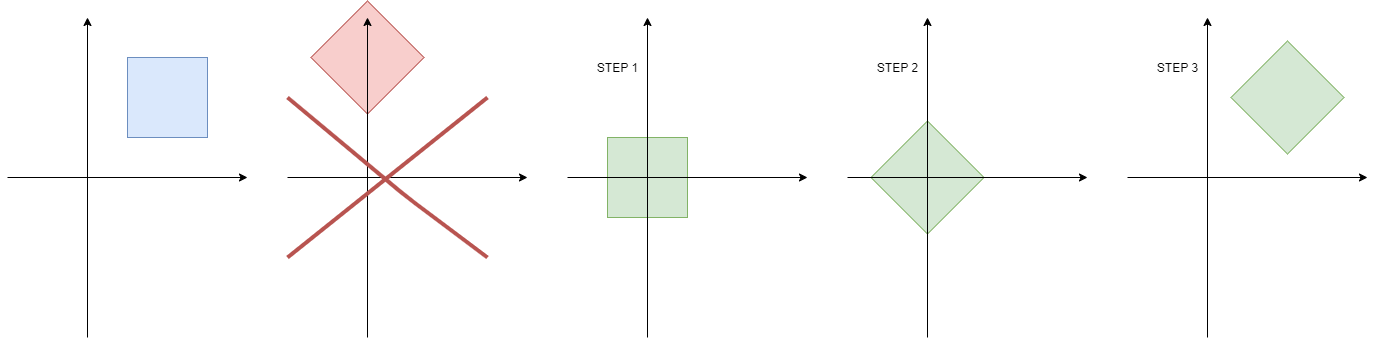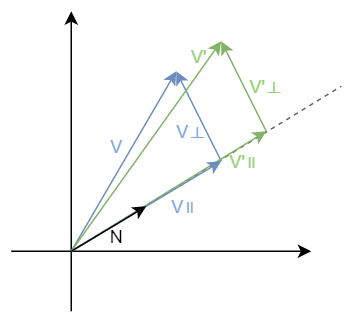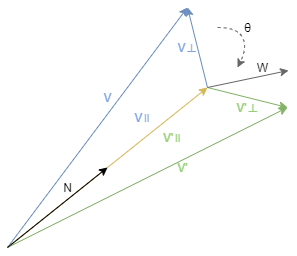# 如何利用矩阵实现平移、缩放、旋转等 3D 变换

## 组合变换和逆变换

CSS3 transform 和 canvas 背后不为人知的秘密 文章的最后介绍了这些 2D 变换如何用矩阵形式表示，可能有同学要问了，为啥非要用矩阵来表示这些变换，之前不挺好的吗？

newPosition = B * (A * position)
= (B * A) * position
= C * position


\begin{aligned} C&=B*A \ &=\begin{bmatrix} 1 & 0 & dx \ 0 & 1 & dy \ 0 & 0 & 1 \end{bmatrix} \begin{bmatrix} cos(\theta) & -sin(\theta) & 0 \ sin(\theta) & cos(\theta) & 0 \ 0 & 0 & 1 \end{bmatrix} \ &=\begin{bmatrix} cos(\theta) & -sin(\theta) & dx \ sin(\theta) & cos(\theta) & dy \ 0 & 0 & 1 \end{bmatrix} \end{aligned}

### 逆变换

$$F^{-1}(F(a)) = F(F^{-1}(a)) = a$$

$$matrix = \begin{bmatrix} 1 & 0 & dx \ 0 & 1 & dy \ 0 & 0 & 1 \end{bmatrix}$$
$$invertMatrix = \begin{bmatrix} 1 & 0 & -dx \ 0 & 1 & -dy \ 0 & 0 & 1 \end{bmatrix}$$

### 绕中心旋转1. 首先我们可以用平移矩阵 $T$ 将物体移动到原点。
2. 再使用旋转矩阵 $R$ 旋转物体
3. 最后使用第一次平移矩阵的逆矩阵 $T^{-1}$ 将物体移回原处

$$T = \begin{bmatrix} 1 & 0 & -dx \ 0 & 1 & -dy \ 0 & 0 & 1 \end{bmatrix}$$
$$R = \begin{bmatrix} cos(\theta) & -sin(\theta) & 0 \ sin(\theta) & cos(\theta) & 0 \ 0 & 0 & 1 \end{bmatrix}$$
$$T^{-1} = \begin{bmatrix} 1 & 0 & dx \ 0 & 1 & dy \ 0 & 0 & 1 \end{bmatrix}$$

$$\begin{bmatrix} cos(\theta) & -sin(\theta) & dx \ sin(\theta) & cos(\theta) & dy \ 0 & 0 & 1 \end{bmatrix}$$

\begin{aligned} M&=T^{-1}RT \ &=\begin{bmatrix} cos(\theta) & -sin(\theta) & -dx * cos(\theta) + dy * sin(\theta) + dx \ sin(\theta) & cos(\theta) & -dx * sin(\theta) -dy * cos(\theta)+dy \ 0 & 0 & 1 \end{bmatrix} \end{aligned}

## 3D 平移矩阵

3D 平移矩阵和 2D 一样，这里不做过多介绍

$$\begin{bmatrix} 1 & 0 & 0 & dx \ 0 & 1 & 0 & dy \ 0 & 0 & 1 & dz \ 0 & 0 & 0 & 1 \end{bmatrix}$$

## 缩放矩阵

3D 缩放矩阵也和 2D 的一样，这里也不做过多介绍。

$$\begin{bmatrix} sx & 0 & 0 & 0 \ 0 & sy & 0 & 0 \ 0 & 0 & sz & 0 \ 0 & 0 & 0 & 1 \end{bmatrix}$$

### 任意方向缩放$$V=V| + V\bot$$
$$V| = (V \cdot N) * N$$
\begin{aligned} V’\bot &= V\bot \ &= V-V| \ &= V-(V \cdot N) * N \end{aligned}
\begin{aligned} V’| &= kV| \ &= k * (V \cdot N) * N \end{aligned}
\begin{aligned} V’ &= V’\bot + V’| \ &= V – (V \cdot N) * N + k * (V \cdot N) * N \ &= V + (k – 1) * (V \cdot N) * N \end{aligned}

$$\begin{bmatrix} 1+(k-1)N_x^2 & (k-1)N_xN_y & (k-1)N_xN_z & 0 \ (k-1)N_xN_y & 1+(k-1)N_y^2 & (k-1)N_xN_z & 0 \ (k-1)N_xN_z & (k-1)N_yN_z & 1+(k-1)N_z^2 & 0 \ 0 & 0 & 0 & 1 \end{bmatrix}$$

## 旋转矩阵

$$R_x = \begin{bmatrix} 1 & 0 & 0 & 0 \ 0 & cos(\theta) & -sin(\theta) & 0 \ 0 & sin(\theta) & cos(\theta) & 0 \ 0 & 0 & 0 & 1 \end{bmatrix}$$

$$R_y = \begin{bmatrix} cos(\theta) & 0 & sin(\theta) & 0 \ 0 & 1 & 0 & 0 \ -sin(\theta) & 0 & cos(\theta) & 0 \ 0 & 0 & 0 & 1 \end{bmatrix}$$

$$R_z = \begin{bmatrix} cos(\theta) & -sin(\theta) & 0 & 0 \ sin(\theta) & cos(\theta) & 0 & 0 \ 0 & 0 & 1 & 0 \ 0 & 0 & 0 & 1 \end{bmatrix}$$

class Mat4 {
return [
1, 0, 0, 0,
0, c, s, 0,
0, -s, c, 0,
0, 0, 0, 1
]
}
return [
c, 0, -s, 0,
0, 1, 0, 0,
s, 0, c, 0,
0, 0, 0, 1
]
}
return [
c, s, 0, 0,
-s, c, 0, 0,
0, 0, 1, 0,
0, 0, 0, 1
]
}
}


https://code.juejin.cn/pen/7168479330178170888

### 绕任意过原点轴旋转$W$、 $V\bot$ 、 $V’\bot$ 都在一个平面上，并且 $W$ 与 $V\bot$ 垂直，我们把 $W$ 和 $V\bot$ 当成水平和垂直坐标轴，根据上方讲到的二维旋转，我们可以得到。

$$V’\bot=cos(\theta)*V\bot + sin(\theta)*W$$

$$V| =(V \cdot N)N$$
\begin{aligned} V\bot &= V-V| \ &=V-(V \cdot N)N \end{aligned}
\begin{aligned} W &= N \times V\bot \ &=N \times (V – V|) \ &=N \times V – N \times V| \ &=N \times V \end{aligned}
\begin{aligned} V’ &= V’\bot + V’| \ &=cos(\theta) * V\bot + sin(\theta) * W + (V \cdot N) * N \ &=cos(\theta) * (V – (V \cdot N) * N) + sin(\theta) * (N \times V) + (V \cdot N) * N \end{aligned}

$$\begin{bmatrix} N_x^2(1-cos(\theta))+cos(\theta) & N_xN_y(1-cos(\theta))-N_zsin(\theta) & N_xN_z(1-cos(\theta))+N_ysin(\theta) & 0 \ N_xN_y(1-cos(\theta))+N_zsin(\theta) & N_y^2(1-cos(\theta))+cos(\theta) & N_yN_z(1-cos(\theta))-N_xsin(\theta) & 0 \ N_xN_z(1-cos(\theta))-N_ysin(\theta) & N_yN_z(1-cos(\theta))+N_xsin(\theta) & N_z^2(1-cos(\theta))+cos(\theta) & 0 \ 0 & 0 & 0 & 1 \end{bmatrix}$$

## 总结

$$F(a+b) = F(a) + F(b) \ F(ka) = kF(a)$$